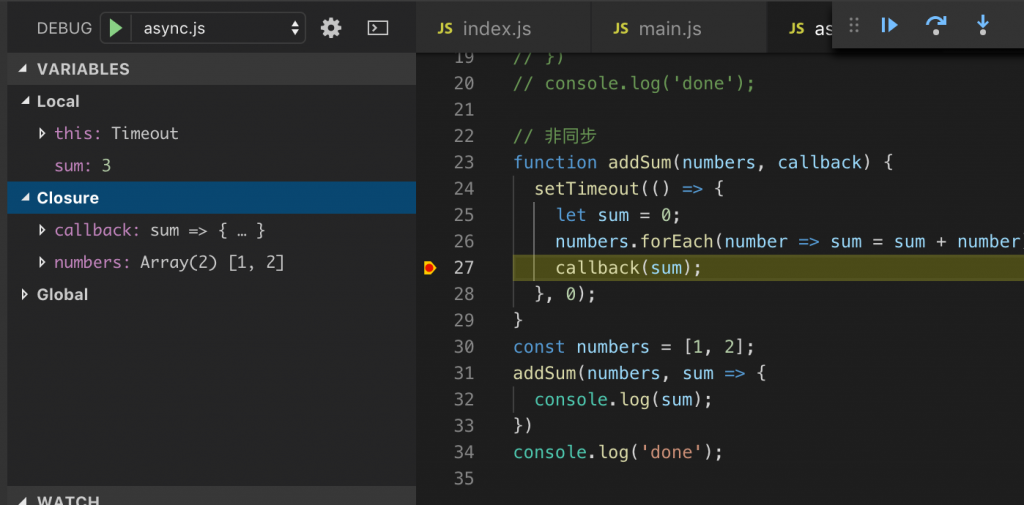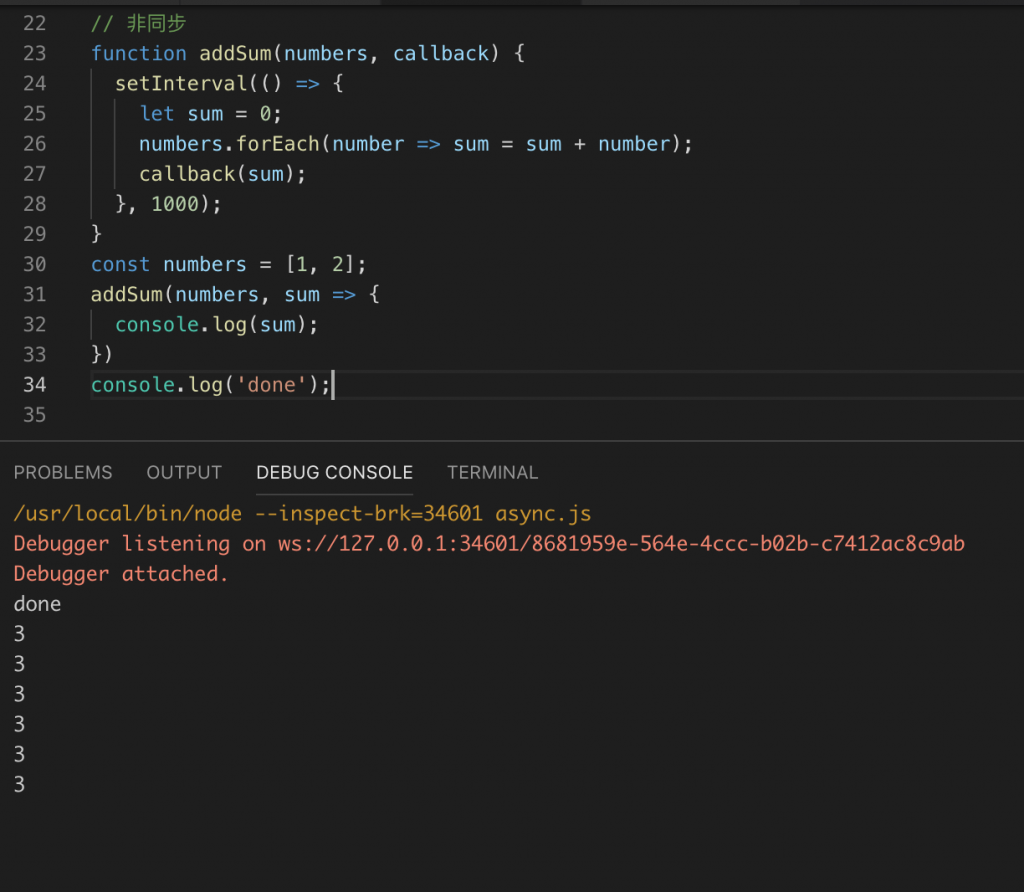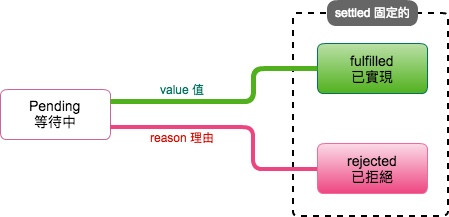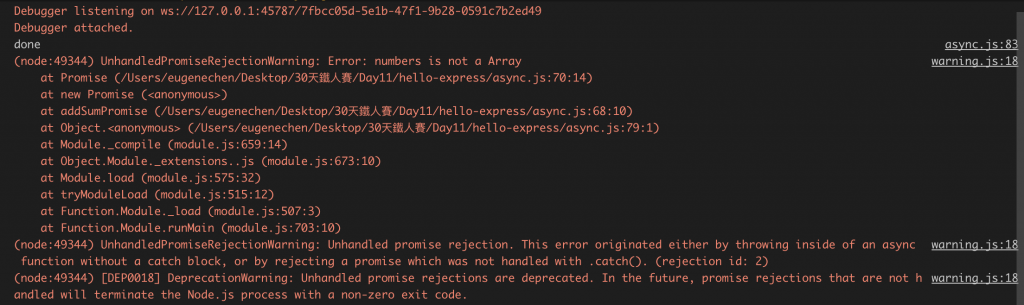#2019 iT 邦幫忙鐵人賽DAY 13
1
Software Development

# 自己做的非同步執行的函數：由同步轉成非同步

1. 考慮以下同步函數，`addSum` 是累加函數

``````// 同步
let sum = 0;
numbers.forEach(number => sum = sum + number);
return sum;
}
const numbers = [1, 2];
``````
2. 先想介面：怎麼回傳？透過一個 callback function

``````// 同步
let sum = 0;
numbers.forEach(number => sum = sum + number);
callback(sum);
}
const numbers = [1, 2];
console.log(sum);
})
console.log('done');
``````

結果：

``````3
done
``````

`addSum(numbers, callback)`看起來是不是有非同步的影子了阿。但他其實還是同步函數，只是在內部呼叫 `callback(sum)`

3. 調用系統計時器 `setTimeout()`

``````// 非同步
setTimeout(() => {
let sum = 0;
numbers.forEach(number => sum = sum + number);
callback(sum);
}, 0);
}
const numbers = [1, 2];
console.log(sum);
})
console.log('done');
``````

結果：

``````done
3
``````

雖然我們設定 0 秒，但不是指馬上執行，`setTimeout()` 會把裡面的 callback 放到 event loop 中的 queue (見 Day 12 - 二周目 - 準備起程深入後端)。當 `addSum()` 執行完後，`console.log('done')`印出 `done`，然後 event loop 就再迴圈一次，重新執行所有 queue 中的 callback function，發現我們有送入

``````() => {
let sum = 0;
numbers.forEach(number => sum = sum + number);
callback(sum);
}
``````

就開始執行，`callback` 這變數因為箭頭函數產生閉包 (見：Day 5 - 一周目- 從VSCode debug 模式看作用域(Scope)、this、閉包(Closure))，如下圖：`callback`有值，值是我們送入的

``````sum => {
console.log(sum);
}
``````

所以 `callback(sum)` 最後印出 `3`1. 詳解 setTimeout、setImmediate、process.nextTick 的區別
2. Node探秘之事件循環（2）--setTimeout/setImmediate/process.nextTick的差別

# 包裝非同步函數

1. 使用計時器(timer)函式: `setTimeout`, `setInterval`
2. 特殊的函式: `nextTick`, `setImmediate`
3. 執行I/O: 監聽網路、資料庫查詢或讀寫外部資源
4. 訂閱事件：常用 `on('event name', callback)` 訂閱

1,2 常用來引起非同步，而 3,4 常用需要包裝非同步函數，使用我們的後端更有可讀性。

## 包裝神器 `Promise`

`Promise` 是一個物件，它遵從Promises/A+標準，下圖截錄自 Promises/A+標準定義Promise 建立時是 pending 狀態，它可收到 value (透過`resolve(value)`) 就被鎖定成 fulfilled，不然就是收到 reason (透過`reject(reason)`, `reason` 一般是 `Error` 物件)就被鎖定成 rejected。鎖定後再也動不了。

`Promise`物件的建立方法如下：

``````new Promise((resolve, reject) => {
});
``````

`(resolve, reject) => {}` 這是 Promise 物件建立時就要立刻傳入的東西，也會立刻執行。

### 用 Promse 包裝 `addSum(number, callback)`：改變函數簽章 `addSumPromise(numbers)`

``````// 包裝非同步回傳 Promise
setTimeout(() => {
let sum = 0;
numbers.forEach(number => sum = sum + number);
callback(sum);
}, 0);
}
return new Promise((resolve, reject) => {
resolve(sum);
})
});
}
const numbers = [1, 2];
.then(sum => {
console.log(sum);
})
console.log('done');
``````

### 強化 `addSumPromise()` 參數處理

``````// 包裝非同步回傳 Promise
setTimeout(() => {
let sum = 0;
numbers.forEach(number => sum = sum + number);
callback(sum);
}, 0);
}
return new Promise((resolve, reject) => {
if(!Array.isArray(numbers)) {
reject(new Error('numbers is not a Array'));
return; // 這行要寫，雖然Promise 狀態不變，但下面的程式一樣會執行
}
resolve(sum);
})
});
}
const numbers = {};
.then(sum => {
console.log(sum);
})
console.log('done');
```````addSumPromise({})` 會得到一個 reject promise。我們注意以下幾點：

1. `console.log('done')` 一樣有執行，不會因為 reject promise。
2. 新版的Node.js 現在會告訢你有一個未處理的 Promise，且這 Error 不是例外發生，不會引起程式插斷(interrupts)，執行會一直下去。因此，你不處理的話就這錯誤就不見了。

``````addSumPromise(numbers)
.then(sum => {
console.log(sum);
})
.catch(error => {
console.error(error);
})
``````

## `then().catch()` 鍊式語法：Promise 物件好用之處

`then().catch()` 可以說是 Promise 的核心之一，`then()``catch()`函數被 Promises/A+ 規定要回傳 Promise，他可以讓我們的非同步操作串接起來。

### `then(callback)`/`catch(callback)`的 callback 叫起與回傳

`then(callback)`/`catch(callback)` 中的 callback 被叫起：

1. `aPromise.then(callback)`：當 aPromise resolve時，resolve value 送入 callback(value)
2. `aPromise.catch(callback)`：當 aPromise reject，reject reason 送入 callback(reason)

`then(callback)`/`catch(callback)` 中的 callback 可以回傳：

1. 回傳 Primitive值：如undefined, object, number, string...等，`then()` 會回傳 resolve promise
``````  .then(() => ({name: 'Billy'})) // resolve promise
``````
2. 回傳 Promise：`then()`會和回傳的 promise 同樣狀態
``````  .then(() => Promise.resolve()) // resolve promise
``````
``````  .then(() => Promise.reject()) // reject promise
``````

### 一個鍊式例子

1. `fetchPerson(name)`　－　查人
2. `fetchOrders(person)`　－　查此人的訂單
``````// then()　鍊式
function fetchOrders(person) {
const orders = person.orderIds.map(id => ({ id }));
return Promise.resolve(orders); // 直接回傳 reolve promise 物件
}

function fetchPerson(name) {
// return Promise.reject(new Error('name is not string')); // 直接回傳 reject promise 物件
return Promise.resolve({
name,
orderIds: ['A', 'B']
});
}

fetchPerson('Billy')
.then(fetchOrders)
.then(orders => {
orders.forEach(order => {
console.log(order);
})
})
.catch(console.error);
``````### `.catch()`常犯的錯：`.catch(callback)` 可能是回傳 resolve promise

``````Promise.resolve(1)
.then(() => Promise.reject(new Error('error 1')))
.catch(console.error)
.then(() => Promise.resolve(2))
.then(console.log)
.catch(console.error)
``````

``````Error: error 1
2
``````

``````function catch(error) => {
return console.error(error); // console.error() 回傳 undefined
}
``````

``````Promise.resolve(1)
.then(() => Promise.reject(new Error('error 1')))
.catch(error => {
console.error(error);
return Promise.reject(error);
})
.then(() => Promise.resolve(2))
.then(console.log)
.catch(console.error)
``````

``````Error: error 1
Error: error 1
``````

### Promise 的例發發生會導致 reject

``````// Promise 的例外發生
function somePromise() {
return new Promise((resolve, reject) => {
// throw new Error('constructor error');
resolve();
});
}
somePromise()
.then(sum => {
// throw new Error('resolve error');
console.log('done');
})
.catch(error => {
console.error(error);
});
``````